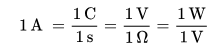# Solar Energy# AmpereThe ampere (symbol: A) is the base unit SI that is used to measure the intensity of the electric current.

It takes its name from the French physicist André-Marie Ampère (1775-1836), one of the leading scholars of electromagnetism.

Being one of the seven basic SI units, all other electromagnetic units are derived from it. By definition, an ampere is the intensity of an electric current that, if maintained in two parallel linear conductors of infinite length and negligible cross-section, placed one meter apart from each other in a vacuum, produces an equal force between them to 2 × 10 -7 N for each meter in length.

The correct way to write amperes is with the initial lowercase letter, except when according to the grammatical rules a word must be written with the initial use of capital letters.

The amp is important, therefore, in the field of electricityThis unit appears in the calculation of the dimensioning of a solar photovoltaic installation.

## Definition of ampere

### Definition of ampere based on electrical phenomena

The ampere expresses the intensity of the current in a conductor crossed in any section from the charge of a coulomb in the time of one second.

By analogy, the intensity of the current is comparable to the amount of water that passes through a tube measured in kg / second, where the mass of water represents the electric chargeThe latter, that is, the amount of electrons, can be expressed in ampere-hours (Ah), which is the total amount of charge that flows, with the intensity of an amperage, in a conductor in an hour. With such a size, for example, the maximum load accumulated by the batteries is measured: the car battery contains approximately 55 Ah.

Within the SI units, equality applies:With "C" for coulomb, "s" for second, "V" for volts, "Ω" for ohms and "W" for wattsThe absence of coefficients is due to the fact that the International System is a coherent measurement system, which means that the product or quotient of several units gives rise to a new unity unit value.

### Definition of ampere based on magnetic phenomena

According to the law of Ampere, the ampere can be defined as the intensity of current that must flow in two cables of infinite length and is placed at the distance of one meter, so that they are attracted with a force equal to 2 × 10 - 7   N / m.

## Measurement samples

Due to the difficulty in measuring the forces between two conductors, the so-called international ampere or statampere was proposed: defined in terms of silver deposition rate (the international ampere is the intensity of a current that, through a solution of AgNO 3 [Ag monovalent], deposited at the cathode 0.001118 g of silver in a second), is equal to 0.999985 A. This unit of measurement, however, is considered obsolete.

Currently, however, most national metrology institutes use sample and resistance stacks for the maintenance of primary amperage samples. The electric current sample is derived from Ohm's law using two samples, one emf and one resistance. In Italy, both samples are kept at INRIM in Turin. The emf sample is a group of saturated heaps of Weston, controlled by the Josephson effect; the electrical resistance is defined as the average resistance of a group of 10 sample resistors in 1 Ω manganin. In order to avoid the influence of the contact resistance between the resistor and the measuring circuit, the sample resistors exhibit a particular four-terminal embodiment,

valoración: 3 - votos 1

Last review: September 7, 2018

Back